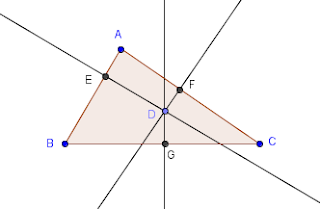GeoGebra Tutorial: Using the Perpendicular Line and the Point on Object Tools

In this tutorial, we use GeoGebra to explore the minimum sum of the distances of a point on the interior of a triangle to its sides. We learn how to use the Point on Object tool and the Perpendicular Line tool. We also learn how to compute using the Input bar. The final output of this tutorial is shown in the following applet.
This is a Java Applet created using GeoGebra from www.geogebra.org – it looks like you don’t have Java installed, please go to www.java.com
Steps in Creating the Applet

 No Instruction 1Using the Polygon tool, construct triangle ABC. 2Select the Point on Object tool, and then click the interior of the triangle to create point D. 3Move point D and observe what happens. Can you move point D outside the triangle? 4Next, we create a line perpendicular to AB passing through D.  To do this, select the Perpendicular Line tool, select segment AB, and then select point D.5Create two more lines passing through D, one perpendicular to AC and the other perpendicular to BC. 6Using the Intersect Two Objects tool, intersect the lines and the perpendicular segments. Your drawing should look like the figure below.7 Hide the three lines by right clicking each line and clicking them and selecting Show Objects. 8Using the Segment between Two Points tool, construct segments DE, DG, and DF.9 To find the total distance t of points D from E, F, and G, type t = g + h + i, and then press the ENTER key. 10Move the points to explore and observe the value of t.

Application

A building is going to be constructed with a triangle bounded by the roads connecting Buildings A, B, and C. A connecting pathway is to be constructed from the three roads to the building. Find the location of the building that will minimize the cost of pathway construction.